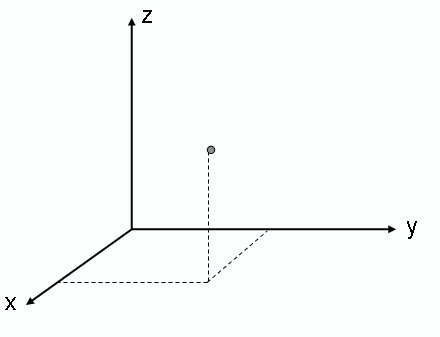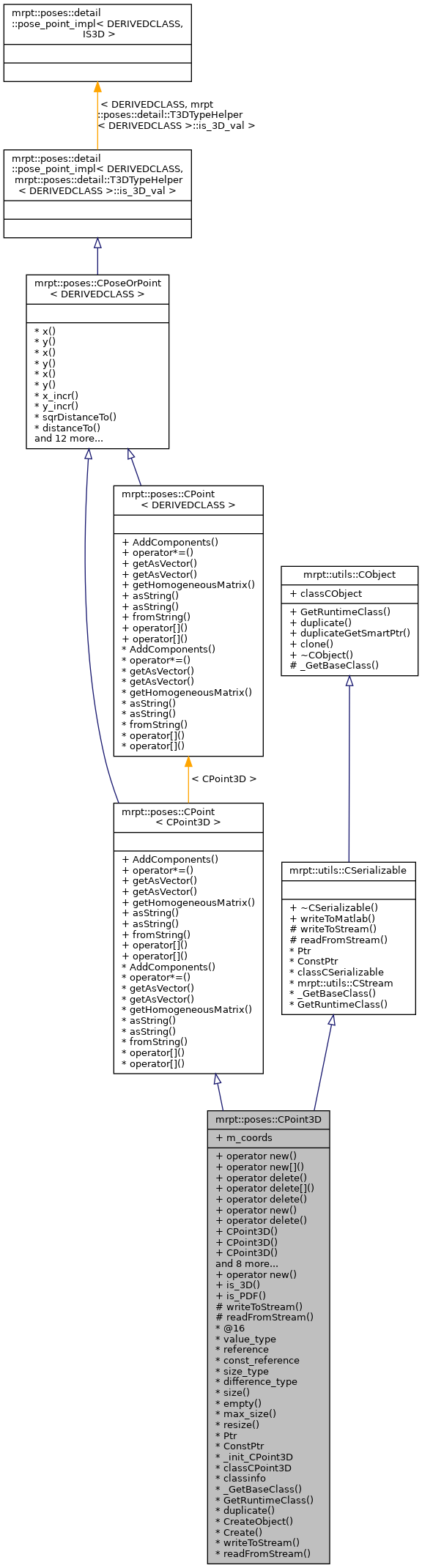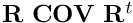Main MRPT website > C++ reference for MRPT 1.5.6
mrpt::poses::CPoint3D Class Reference

Detailed Description

A class used to store a 3D point.

For a complete description of Points/Poses, see mrpt::poses::CPoseOrPoint, or refer to the 2D/3D Geometry tutorial in the wiki.CPoseOrPoint,CPose, CPoint

Definition at line 32 of file CPoint3D.h.

#include <mrpt/poses/CPoint3D.h>

Inheritance diagram for mrpt::poses::CPoint3D:Public Types

enum  { is_3D_val = 1 }

enum  { is_PDF_val = 0 }

Public Member Functions

voidoperator new (size_t size)

voidoperator new[] (size_t size)

void operator delete (void *ptr) throw ()

void operator delete[] (void *ptr) throw ()

void operator delete (void *memory, void *ptr) throw ()

voidoperator new (size_t size, const std::nothrow_t &) throw ()

void operator delete (void *ptr, const std::nothrow_t &) throw ()

CPoint3D (const double x=0, const double y=0, const double z=0)
Constructor for initializing point coordinates. More...

CPoint3D (const mrpt::math::CArrayDouble< 3 > &xyz)
Constructor from a XYZ 3-vector. More...

CPoint3D (const CPoint2D &p)
Constructor from an CPoint2D object. More...

CPoint3D (const CPose3D &p)
Constructor from an CPose3D object. More...

CPoint3D (const CPose2D &p)
Constructor from an CPose2D object. More...

CPoint3D (const mrpt::math::TPoint3D &p)
Constructor from lightweight object. More...

CPoint3D operator- (const CPose3D &b) const
Returns this point as seen from "b", i.e. More...

CPoint3D operator- (const CPoint3D &b) const
Returns this point minus point "b", i.e. More...

CPoint3D operator+ (const CPoint3D &b) const
Returns this point plus point "b", i.e. More...

CPose3D operator+ (const CPose3D &b) const
Returns this point plus pose "b", i.e. More...

void setToNaN () MRPT_OVERRIDE
Set all data fields to quiet NaN. More...

virtual mxArraywriteToMatlab () const
Introduces a pure virtual method responsible for writing to a mxArray Matlab object, typically a MATLAB struct whose contents are documented in each derived class. More...

mrpt::utils::CObjectPtr duplicateGetSmartPtr () const
Returns a copy of the object, indepently of its class, as a smart pointer (the newly created object will exist as long as any copy of this smart pointer). More...

CObjectclone () const
Cloning interface for smart pointers. More...

Methods common to all 2D or 3D points
Scalar addition of all coordinates. More...

void operator*= (const double s)
Scalar multiplication. More...

void getAsVector (mrpt::math::CVectorDouble &v) const
Return the pose or point as a 1x2 or 1x3 vector [x y] or [x y z]. More...

mrpt::math::CVectorDouble getAsVector () const

void getHomogeneousMatrix (mrpt::math::CMatrixDouble44 &out_HM) const
Returns the corresponding 4x4 homogeneous transformation matrix for the point(translation) or pose (translation+orientation). More...

void asString (std::string &s) const
Returns a human-readable textual representation of the object (eg: "[0.02 1.04]" ) More...

std::string asString () const

void fromString (const std::string &s)
Set the current object value from a string generated by 'asString' (eg: "[0.02 1.04]" ) More...

const double & operator[] (unsigned int i) const

double & operator[] (unsigned int i)

Static Public Member Functions

static voidoperator new (size_t size, void *ptr)

static bool is_3D ()

static bool is_PDF ()

Public Attributes

mrpt::math::CArrayDouble< 3 > m_coords
[x,y,z] More...

Static Public Attributes

static const
mrpt::utils::TRuntimeClassId
classCObject

RTTI stuff
static const
mrpt::utils::TRuntimeClassId
classCSerializable

Protected Member Functions

CSerializable virtual methods
void writeToStream (mrpt::utils::CStream &out, int *getVersion) const
Introduces a pure virtual method responsible for writing to a CStream. More...

void readFromStream (mrpt::utils::CStream &in, int version)
Introduces a pure virtual method responsible for loading from a CStream This can not be used directly be users, instead use "stream >> object;" for reading it from a stream or "stream >> object_ptr;" if the class is unknown apriori. More...

RTTI stuff

typedef CPoint3DPtr Ptr

typedef CPoint3DPtr ConstPtr

static mrpt::utils::CLASSINIT _init_CPoint3D

static mrpt::utils::TRuntimeClassId classCPoint3D

static const
mrpt::utils::TRuntimeClassId
classinfo

static const
mrpt::utils::TRuntimeClassId
_GetBaseClass ()

virtual const
mrpt::utils::TRuntimeClassId
GetRuntimeClass () const
Returns information about the class of an object in runtime. More...

virtual mrpt::utils::CObjectduplicate () const
Returns a copy of the object, indepently of its class. More...

static mrpt::utils::CObjectCreateObject ()

static CPoint3DPtr Create ()

STL-like methods and typedefs

enum  { static_size = 3 }

typedef double value_type
The type of the elements. More...

typedef double & reference

typedef const double & const_reference

typedef std::size_t size_type

typedef std::ptrdiff_t difference_type

static size_type size ()

static bool empty ()

static size_type max_size ()

static void resize (const size_t n)

double x () const
Common members of all points & poses classes. More...

double & x ()

void x (const double v)

double y () const

double & y ()

void y (const double v)

void x_incr (const double v)

void y_incr (const double v)

double sqrDistanceTo (const CPoseOrPoint< OTHERCLASS > &b) const
Returns the squared euclidean distance to another pose/point: More...

double distanceTo (const CPoseOrPoint< OTHERCLASS > &b) const
Returns the Euclidean distance to another pose/point: More...

double distanceTo (const mrpt::math::TPoint3D &b) const
Returns the euclidean distance to a 3D point: More...

double distance2DToSquare (double ax, double ay) const
Returns the squared 2D distance from this pose/point to a 2D point (ignores Z, if it exists). More...

double distance3DToSquare (double ax, double ay, double az) const
Returns the squared 3D distance from this pose/point to a 3D point. More...

double distance2DTo (double ax, double ay) const
Returns the 2D distance from this pose/point to a 2D point (ignores Z, if it exists). More...

double distance3DTo (double ax, double ay, double az) const
Returns the 3D distance from this pose/point to a 3D point. More...

double norm () const
Returns the euclidean norm of vector:. More...

mrpt::math::CVectorDouble getAsVectorVal () const
Return the pose or point as a 1xN vector with all the components (see derived classes for each implementation) More...

mrpt::math::CMatrixDouble44 getHomogeneousMatrixVal () const
Returns the corresponding 4x4 homogeneous transformation matrix for the point(translation) or pose (translation+orientation). More...

void getInverseHomogeneousMatrix (mrpt::math::CMatrixDouble44 &out_HM) const
Returns the corresponding 4x4 inverse homogeneous transformation matrix for this point or pose. More...

mrpt::math::CMatrixDouble44 getInverseHomogeneousMatrix () const

static bool is3DPoseOrPoint ()
Return true for poses or points with a Z component, false otherwise. More...

Member Typedef Documentation

 typedef const double& mrpt::poses::CPoint3D::const_reference

Definition at line 82 of file CPoint3D.h.

 typedef CPoint3DPtr mrpt::poses::CPoint3D::ConstPtr

Definition at line 35 of file CPoint3D.h.

Definition at line 84 of file CPoint3D.h.

 typedef CPoint3DPtr mrpt::poses::CPoint3D::Ptr

A typedef for the associated smart pointer

Definition at line 35 of file CPoint3D.h.

 typedef double& mrpt::poses::CPoint3D::reference

Definition at line 81 of file CPoint3D.h.

 typedef std::size_t mrpt::poses::CPoint3D::size_type

Definition at line 83 of file CPoint3D.h.

 typedef double mrpt::poses::CPoint3D::value_type

The type of the elements.

Definition at line 80 of file CPoint3D.h.

Member Enumeration Documentation

 anonymous enum
Enumerator
is_3D_val

Definition at line 72 of file CPoint3D.h.

 anonymous enum
Enumerator
is_PDF_val

Definition at line 75 of file CPoint3D.h.

 anonymous enum
Enumerator
static_size

Definition at line 88 of file CPoint3D.h.

Constructor & Destructor Documentation

 mrpt::poses::CPoint3D::CPoint3D ( const double x = 0, const double y = 0, const double z = 0 )
inline

Constructor for initializing point coordinates.

Definition at line 42 of file CPoint3D.h.

 mrpt::poses::CPoint3D::CPoint3D ( const mrpt::math::CArrayDouble< 3 > & xyz )
inlineexplicit

Constructor from a XYZ 3-vector.

Definition at line 45 of file CPoint3D.h.

 CPoint3D::CPoint3D ( const CPoint2D & p )
explicit

Constructor from an CPoint2D object.

Definition at line 27 of file CPoint3D.cpp.

 CPoint3D::CPoint3D ( const CPose3D & p )
explicit

Constructor from an CPose3D object.

Definition at line 32 of file CPoint3D.cpp.

 CPoint3D::CPoint3D ( const CPose2D & p )
explicit

Constructor from an CPose2D object.

Definition at line 29 of file CPoint3D.cpp.

 mrpt::poses::CPoint3D::CPoint3D ( const mrpt::math::TPoint3D & p )
inlineexplicit

Constructor from lightweight object.

Definition at line 57 of file CPoint3D.h.

Member Function Documentation

 static const mrpt::utils::TRuntimeClassId* mrpt::poses::CPoint3D::_GetBaseClass ( )
staticprotected
 void mrpt::poses::CPoint< CPoint3D >::AddComponents ( const OTHERCLASS & b )
inlineinherited

This is diferent from poses/point composition, which is implemented as "+" operators in classes derived from "CPose"

Definition at line 36 of file CPoint.h.

References min, and static_size.

 void mrpt::poses::CPoint< CPoint3D >::asString ( std::string & s ) const
inlineinherited

Returns a human-readable textual representation of the object (eg: "[0.02 1.04]" )

fromString

Definition at line 75 of file CPoint.h.

 std::string mrpt::poses::CPoint< CPoint3D >::asString ( ) const
inlineinherited

Definition at line 81 of file CPoint.h.

 CObject* mrpt::utils::CObject::clone ( ) const
inlineinherited

Cloning interface for smart pointers.

Definition at line 143 of file CObject.h.

 static CPoint3DPtr mrpt::poses::CPoint3D::Create ( )
static
 static mrpt::utils::CObject* mrpt::poses::CPoint3D::CreateObject ( )
static
 double mrpt::poses::CPoseOrPoint< CPoint3D >::distance2DTo ( double ax, double ay ) const
inlineinherited

Returns the 2D distance from this pose/point to a 2D point (ignores Z, if it exists).

Definition at line 165 of file CPoseOrPoint.h.

 double mrpt::poses::CPoseOrPoint< CPoint3D >::distance2DToSquare ( double ax, double ay ) const
inlineinherited

Returns the squared 2D distance from this pose/point to a 2D point (ignores Z, if it exists).

Definition at line 156 of file CPoseOrPoint.h.

 double mrpt::poses::CPoseOrPoint< CPoint3D >::distance3DTo ( double ax, double ay, double az ) const
inlineinherited

Returns the 3D distance from this pose/point to a 3D point.

Definition at line 168 of file CPoseOrPoint.h.

 double mrpt::poses::CPoseOrPoint< CPoint3D >::distance3DToSquare ( double ax, double ay, double az ) const
inlineinherited

Returns the squared 3D distance from this pose/point to a 3D point.

Definition at line 159 of file CPoseOrPoint.h.

 double mrpt::poses::CPoseOrPoint< CPoint3D >::distanceTo ( const CPoseOrPoint< OTHERCLASS > & b ) const
inlineinherited

Returns the Euclidean distance to another pose/point:

Definition at line 150 of file CPoseOrPoint.h.

 double mrpt::poses::CPoseOrPoint< CPoint3D >::distanceTo ( const mrpt::math::TPoint3D & b ) const
inlineinherited

Returns the euclidean distance to a 3D point:

Definition at line 171 of file CPoseOrPoint.h.

 virtual mrpt::utils::CObject* mrpt::poses::CPoint3D::duplicate ( ) const
virtual

Returns a copy of the object, indepently of its class.

Implements mrpt::utils::CObject.

 mrpt::utils::CObjectPtr mrpt::utils::CObject::duplicateGetSmartPtr ( ) const
inlineinherited

Returns a copy of the object, indepently of its class, as a smart pointer (the newly created object will exist as long as any copy of this smart pointer).

Definition at line 140 of file CObject.h.

 static bool mrpt::poses::CPoint3D::empty ( )
inlinestatic

Definition at line 90 of file CPoint3D.h.

 void mrpt::poses::CPoint< CPoint3D >::fromString ( const std::string & s )
inlineinherited

Set the current object value from a string generated by 'asString' (eg: "[0.02 1.04]" )

asString
Exceptions
 std::exception On invalid format

Definition at line 87 of file CPoint.h.

References ASSERT_EQUAL_, mrpt::mrpt::math::size(), static_size, and THROW_EXCEPTION.

 void mrpt::poses::CPoint< CPoint3D >::getAsVector ( mrpt::math::CVectorDouble & v ) const
inlineinherited

Return the pose or point as a 1x2 or 1x3 vector [x y] or [x y z].

Definition at line 51 of file CPoint.h.

References static_size.

 mrpt::math::CVectorDouble mrpt::poses::CPoint< CPoint3D >::getAsVector ( ) const
inlineinherited

This is an overloaded member function, provided for convenience. It differs from the above function only in what argument(s) it accepts.

Definition at line 58 of file CPoint.h.

 mrpt::math::CVectorDouble mrpt::poses::CPoseOrPoint< CPoint3D >::getAsVectorVal ( ) const
inlineinherited

Return the pose or point as a 1xN vector with all the components (see derived classes for each implementation)

Definition at line 181 of file CPoseOrPoint.h.

 void mrpt::poses::CPoint< CPoint3D >::getHomogeneousMatrix ( mrpt::math::CMatrixDouble44 & out_HM ) const
inlineinherited

Returns the corresponding 4x4 homogeneous transformation matrix for the point(translation) or pose (translation+orientation).

getInverseHomogeneousMatrix

Definition at line 63 of file CPoint.h.

 mrpt::math::CMatrixDouble44 mrpt::poses::CPoseOrPoint< CPoint3D >::getHomogeneousMatrixVal ( ) const
inlineinherited

Returns the corresponding 4x4 homogeneous transformation matrix for the point(translation) or pose (translation+orientation).

getInverseHomogeneousMatrix

Definition at line 191 of file CPoseOrPoint.h.

References mrpt::math::UNINITIALIZED_MATRIX.

 void mrpt::poses::CPoseOrPoint< CPoint3D >::getInverseHomogeneousMatrix ( mrpt::math::CMatrixDouble44 & out_HM ) const
inlineinherited

Returns the corresponding 4x4 inverse homogeneous transformation matrix for this point or pose.

getHomogeneousMatrix

Definition at line 201 of file CPoseOrPoint.h.

References mrpt::math::homogeneousMatrixInverse().

 mrpt::math::CMatrixDouble44 mrpt::poses::CPoseOrPoint< CPoint3D >::getInverseHomogeneousMatrix ( ) const
inlineinherited

This is an overloaded member function, provided for convenience. It differs from the above function only in what argument(s) it accepts.

Definition at line 208 of file CPoseOrPoint.h.

 virtual const mrpt::utils::TRuntimeClassId* mrpt::poses::CPoint3D::GetRuntimeClass ( ) const
virtual

Returns information about the class of an object in runtime.

Reimplemented from mrpt::utils::CSerializable.

 static bool mrpt::poses::CPoseOrPoint< CPoint3D >::is3DPoseOrPoint ( )
inlinestaticinherited

Return true for poses or points with a Z component, false otherwise.

Definition at line 127 of file CPoseOrPoint.h.

 static bool mrpt::poses::CPoint3D::is_3D ( )
inlinestatic

Definition at line 73 of file CPoint3D.h.

 static bool mrpt::poses::CPoint3D::is_PDF ( )
inlinestatic

Definition at line 76 of file CPoint3D.h.

 static size_type mrpt::poses::CPoint3D::max_size ( )
inlinestatic

Definition at line 91 of file CPoint3D.h.

References static_size.

 double mrpt::poses::CPoseOrPoint< CPoint3D >::norm ( ) const
inlineinherited

Returns the euclidean norm of vector:.

Definition at line 174 of file CPoseOrPoint.h.

 void mrpt::poses::CPoint3D::operator delete ( void * ptr ) throw ( )
inline

Definition at line 35 of file CPoint3D.h.

 void mrpt::poses::CPoint3D::operator delete ( void * memory, void * ptr ) throw ( )
inline

Definition at line 35 of file CPoint3D.h.

 void mrpt::poses::CPoint3D::operator delete ( void * ptr, const std::nothrow_t & ) throw ( )
inline

Definition at line 35 of file CPoint3D.h.

 void mrpt::poses::CPoint3D::operator delete[] ( void * ptr ) throw ( )
inline

Definition at line 35 of file CPoint3D.h.

 static void* mrpt::poses::CPoint3D::operator new ( size_t size, void * ptr )
inlinestatic

Definition at line 35 of file CPoint3D.h.

 void* mrpt::poses::CPoint3D::operator new ( size_t size, const std::nothrow_t & ) throw ( )
inline

Definition at line 35 of file CPoint3D.h.

 void* mrpt::poses::CPoint3D::operator new ( size_t size )
inline

Definition at line 35 of file CPoint3D.h.

 void* mrpt::poses::CPoint3D::operator new[] ( size_t size )
inline

Definition at line 35 of file CPoint3D.h.

 void mrpt::poses::CPoint< CPoint3D >::operator*= ( const double s )
inlineinherited

Scalar multiplication.

Definition at line 44 of file CPoint.h.

References static_size.

 CPoint3D CPoint3D::operator+ ( const CPoint3D & b ) const

Returns this point plus point "b", i.e.

result = this + b

Definition at line 104 of file CPoint3D.cpp.

References m_coords.

 CPose3D CPoint3D::operator+ ( const CPose3D & b ) const

Returns this point plus pose "b", i.e.

result = this + b

Definition at line 112 of file CPoint3D.cpp.

 CPoint3D CPoint3D::operator- ( const CPose3D & b ) const

Returns this point as seen from "b", i.e.

result = this - b

Definition at line 78 of file CPoint3D.cpp.

 CPoint3D CPoint3D::operator- ( const CPoint3D & b ) const

Returns this point minus point "b", i.e.

result = this - b

Definition at line 95 of file CPoint3D.cpp.

References m_coords.

 const double& mrpt::poses::CPoint< CPoint3D >::operator[] ( unsigned int i ) const
inlineinherited

Definition at line 97 of file CPoint.h.

 double& mrpt::poses::CPoint< CPoint3D >::operator[] ( unsigned int i )
inlineinherited

Definition at line 98 of file CPoint.h.

 void CPoint3D::readFromStream ( mrpt::utils::CStream & in, int version )
protectedvirtual

Introduces a pure virtual method responsible for loading from a CStream This can not be used directly be users, instead use "stream >> object;" for reading it from a stream or "stream >> object_ptr;" if the class is unknown apriori.

Parameters
 in The input binary stream where the object data must read from. version The version of the object stored in the stream: use this version number in your code to know how to read the incoming data.
Exceptions
 std::exception On any error, see CStream::ReadBuffer
CStream

Implements mrpt::utils::CSerializable.

Definition at line 54 of file CPoint3D.cpp.

References MRPT_THROW_UNKNOWN_SERIALIZATION_VERSION.

 static void mrpt::poses::CPoint3D::resize ( const size_t n )
inlinestatic

Definition at line 92 of file CPoint3D.h.

References mrpt::format(), and static_size.

 void CPoint3D::setToNaN ( )
virtual

Set all data fields to quiet NaN.

Implements mrpt::poses::CPoseOrPoint< CPoint3D >.

Definition at line 117 of file CPoint3D.cpp.

 static size_type mrpt::poses::CPoint3D::size ( )
inlinestatic

Definition at line 89 of file CPoint3D.h.

References static_size.

 double mrpt::poses::CPoseOrPoint< CPoint3D >::sqrDistanceTo ( const CPoseOrPoint< OTHERCLASS > & b ) const
inlineinherited

Returns the squared euclidean distance to another pose/point:

Definition at line 130 of file CPoseOrPoint.h.

 virtual mxArray* mrpt::utils::CSerializable::writeToMatlab ( ) const
inlinevirtualinherited

Introduces a pure virtual method responsible for writing to a mxArray Matlab object, typically a MATLAB struct whose contents are documented in each derived class.

Returns
A new mxArray (caller is responsible of memory freeing) or NULL is class does not support conversion to MATLAB.

Definition at line 79 of file CSerializable.h.

 void CPoint3D::writeToStream ( mrpt::utils::CStream & out, int * getVersion ) const
protectedvirtual

Introduces a pure virtual method responsible for writing to a CStream.

This can not be used directly be users, instead use "stream << object;" for writing it to a stream.

Parameters
 out The output binary stream where object must be dumped. getVersion If NULL, the object must be dumped. If not, only the version of the object dump must be returned in this pointer. This enables the versioning of objects dumping and backward compatibility with previously stored data.
Exceptions
 std::exception On any error, see CStream::WriteBuffer
CStream

Implements mrpt::utils::CSerializable.

Definition at line 39 of file CPoint3D.cpp.

 double mrpt::poses::CPoseOrPoint< CPoint3D >::x ( ) const
inlineinherited

Common members of all points & poses classes.

< Get X coord.

Definition at line 113 of file CPoseOrPoint.h.

 double& mrpt::poses::CPoseOrPoint< CPoint3D >::x ( )
inlineinherited

Definition at line 116 of file CPoseOrPoint.h.

 void mrpt::poses::CPoseOrPoint< CPoint3D >::x ( const double v )
inlineinherited
Parameters
 v Set X coord.

Definition at line 119 of file CPoseOrPoint.h.

 void mrpt::poses::CPoseOrPoint< CPoint3D >::x_incr ( const double v )
inlineinherited
Parameters
 v X+=v

Definition at line 122 of file CPoseOrPoint.h.

 double mrpt::poses::CPoseOrPoint< CPoint3D >::y ( ) const
inlineinherited

< Get Y coord.

Definition at line 114 of file CPoseOrPoint.h.

 double& mrpt::poses::CPoseOrPoint< CPoint3D >::y ( )
inlineinherited

Definition at line 117 of file CPoseOrPoint.h.

 void mrpt::poses::CPoseOrPoint< CPoint3D >::y ( const double v )
inlineinherited
Parameters
 v Set Y coord.

Definition at line 120 of file CPoseOrPoint.h.

 void mrpt::poses::CPoseOrPoint< CPoint3D >::y_incr ( const double v )
inlineinherited
Parameters
 v Y+=v

Definition at line 123 of file CPoseOrPoint.h.

Member Data Documentation

 mrpt::utils::CLASSINIT mrpt::poses::CPoint3D::_init_CPoint3D
staticprotected

Definition at line 35 of file CPoint3D.h.

 const mrpt::utils::TRuntimeClassId mrpt::utils::CObject::classCObject
staticinherited

Definition at line 128 of file CObject.h.

 mrpt::utils::TRuntimeClassId mrpt::poses::CPoint3D::classCPoint3D
static

Definition at line 35 of file CPoint3D.h.

 const mrpt::utils::TRuntimeClassId mrpt::utils::CSerializable::classCSerializable
staticinherited

Definition at line 42 of file CSerializable.h.

 const mrpt::utils::TRuntimeClassId* mrpt::poses::CPoint3D::classinfo
static

Definition at line 35 of file CPoint3D.h.

 mrpt::math::CArrayDouble<3> mrpt::poses::CPoint3D::m_coords

[x,y,z]

Definition at line 38 of file CPoint3D.h.

Referenced by operator+(), mrpt::poses::CPose3DRotVec::operator+(), and operator-().

 Page generated by Doxygen 1.8.6 for MRPT 1.5.6 Git: 4c65e84 Tue Apr 24 08:18:17 2018 +0200 at mar abr 24 08:26:17 CEST 2018